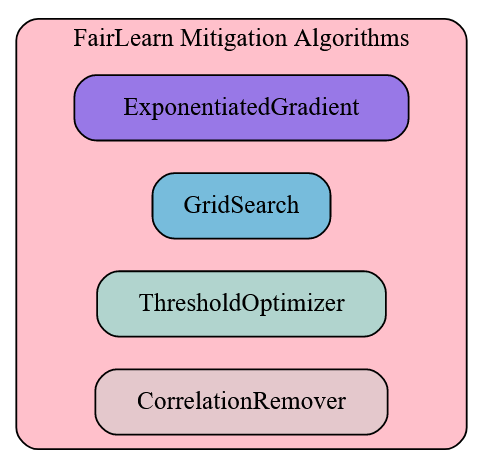So as always, let’s start with a quick recap of what was covered during the session:

TLDR: Covered Chapter 5 of Deep Learning for Coders book, includes secret recipes to build a great ML model.

• We started with PETs dataset. This time we were classifying Breed of Cat/Dog using fastai image classifier.
• The dataset followed a particular heuristics, which represented Species with the capital case as Cats and smaller case as Dogs. For the breeds also a similar pattern is there:

`Path('/root/.fastai/data/oxford-iiit-pet/images/Maine_Coon_157.jpg')`

• The breed can be obtained using the regex patterns. It’s recommended to read a regex tutorial and solve it with a regex problem set. `re.findall(r''(.+)_\d+.jpg)`
• Most functions and methods in fastai return a special collection class called L(part of fastai core library)
``````pets = DataBlock(blocks=(ImageBlock, CategoryBlock),
get_items=get_image_files,
splitter=RandomSplitter(seed=42),
get_y=using_attr(RegexLabeller(r'(.+)_\d+.jpg\$'), 'name'),
item_tfms=Resize(460),
batch_tfms=aug_transforms(size=224, min_scale=0.75)
)
``````

### What is Presizing?

• It’s a technique in which we transform input image into a larger size image, and then use augmentation techniques like Random cropping to get the full picture of what we are trying to classify.
• Usually for transforming images to larger sizes like Resize operation we use a CPU(Central Processing Unit). Then process of augmentation techniques like cropping, RandomResizing is usually done with a GPU(Graphics process units) because it requires uniform size images.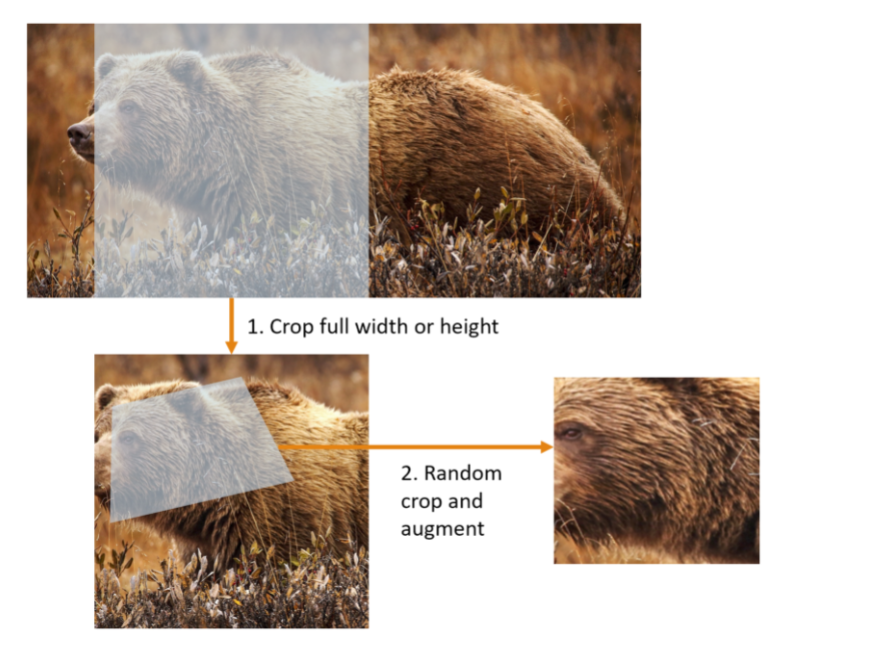• In practice, according to Aman Arora we spend a lot of time loading data correctly when working with a new dataset. This is where some errors come.
• When errors come in our data block, use the `summary method in DataBlock`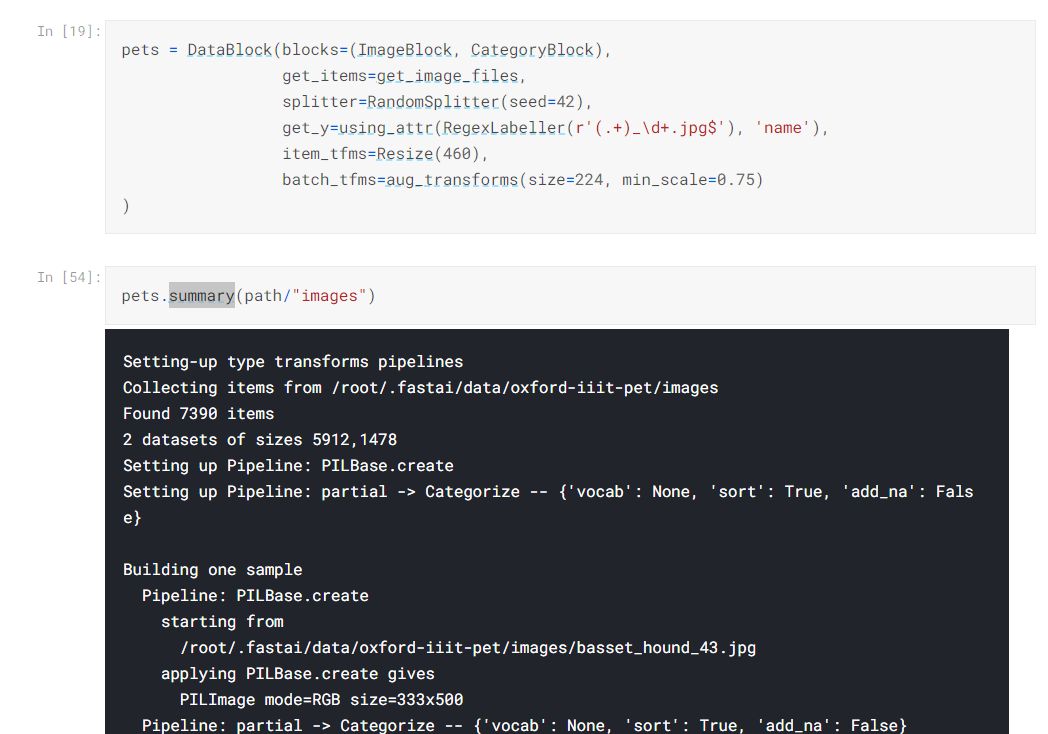• Now when training our model, across a few epochs(doing a complete pass of data). The learn.fine_tune() method shows average loss over the training dataset and loss of validation set. What is this loss function used, is it the loss function we learned in chapter 4?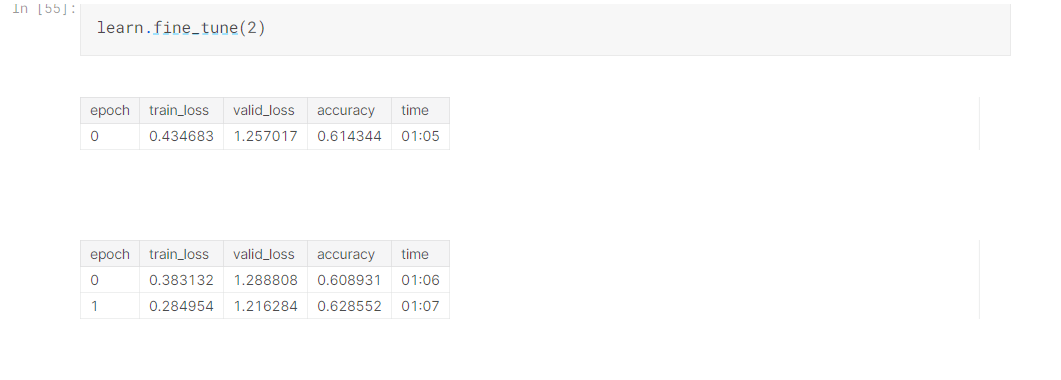• By default, fastai chooses the appropriate loss function based on Datablock type. Generally for an image dataset, with a categorical outcome we usually use cross-entropy loss

### What is Cross Entropy Loss?

Let’s look it step by step. Let’s first view the activations and labels of our data loader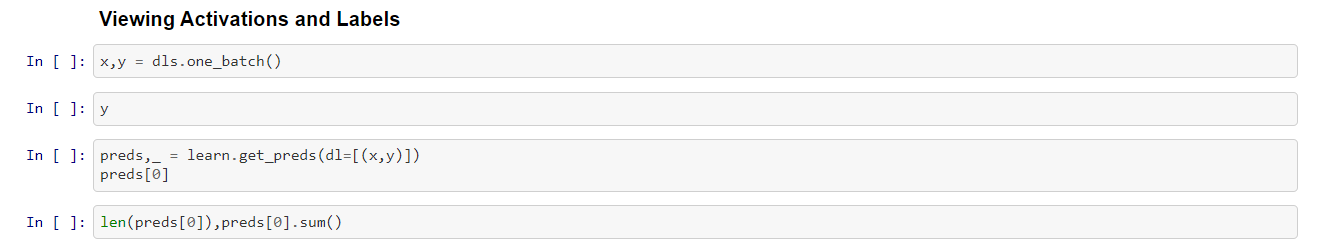• We can see our pets are converted into associated tensor values representing the category into 0-37 values in tensor.
• The prediction probabilities sum all add up to 1

What is Softmax function?

• When we were classifying 3s and 7s in MNIST dataset, it was a binary classification problem, with the target being a boolean value. We used the sigmoid function
• Cross-Entropy loss is being used because of two benefits:
1. It works when our dependant variables(like classifying pet breeds) with more than two categories
2. It results in a faster and reliable training
• Now for classifying 3s and 7s, it is reliable for a set of random activations to be substracted with the difference of activation for three and seven. After calculating the sigmoid function of their difference.

`(acts[:, 0] - acts[:, 1]).sigmoid()`

• The softmax function is represented in the formula as below. Obviously there is a PyTorch function for it
``````def softmax(x):
return (exp(x) /exp(x).sum(dim=1, keepdim=True))
``````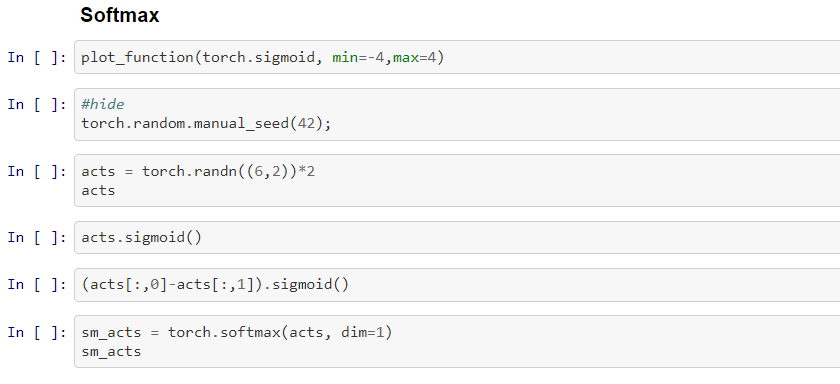What is log-likelihood loss

• We need to calculate the loss for each no like mnist_loss(target=1, 1-inputs). Yet for multiple categories, we need a better method
• For this, we use the log-likelihood loss method. When considering things in log scale, a 99.99% and 99.9% is usually 10 times larger, even though in absolute terms it seems small.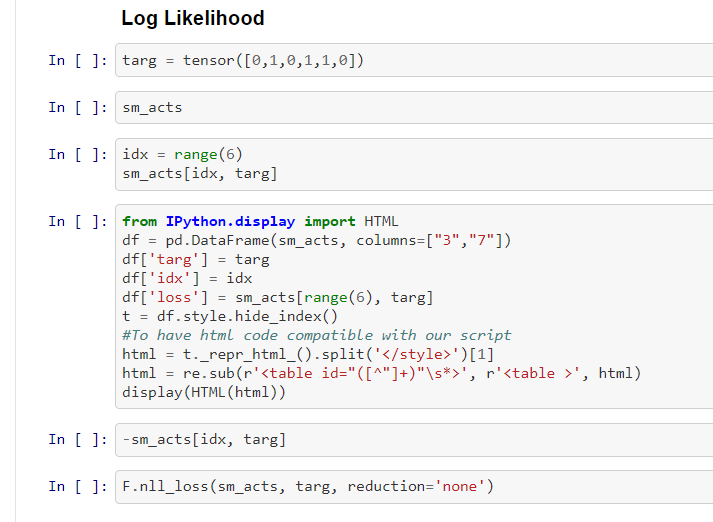Combining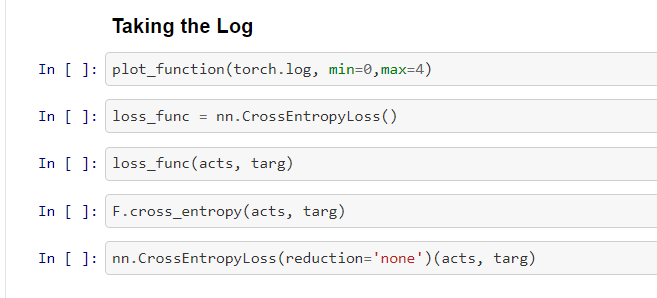• Softmax + Negative Log Likelihood = Cross Entropy Loss
• Most of the time, we calculate with torch methods like `nn.CrossEntropyLoss(reduction='none')(acts, targ)`.

### Model Interpretation result

• The wrong results, and where our model has been wrong can’t be often spotted merely with help of metrics like accuracy.
• So we use a confusion matrix and plot the function with top losses.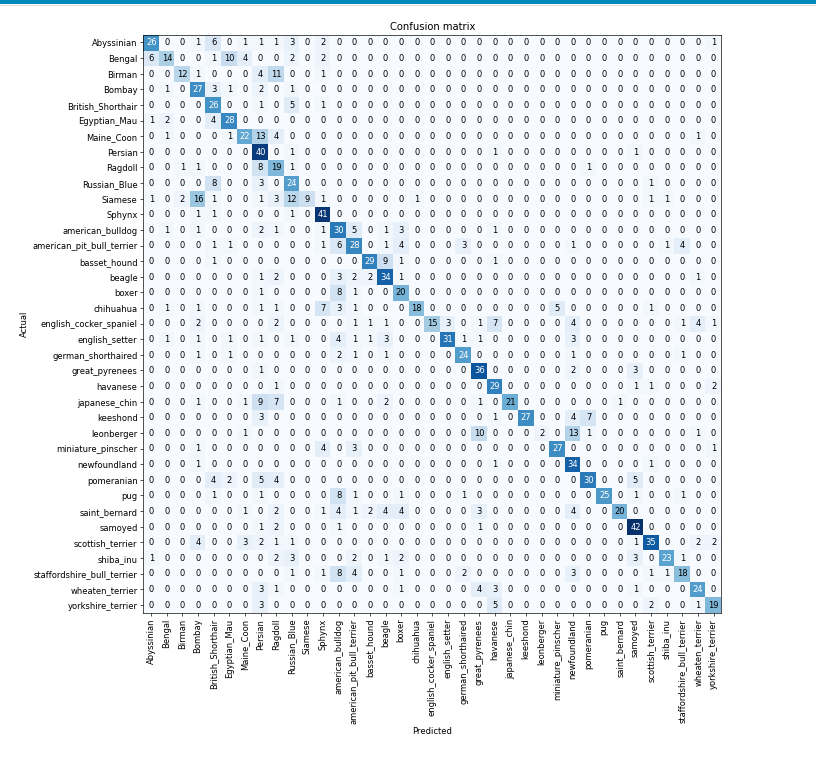### Learning rate finder and freezing pre-trained layers

• Using a learning rate finder developed by Leslie Smith, we can find the minimum step and maximum steep point of learning rate
• To find the appropriate learning rate, using at some point of the middle of lr_rate_finder() is a food idea. But always remember to use logarithmic scale even here to calculate learning rate finder
• When we are using models with transfer learning, they are not trained for the specific model which we are trying to classify.
• For this, we freeze the last layers and train again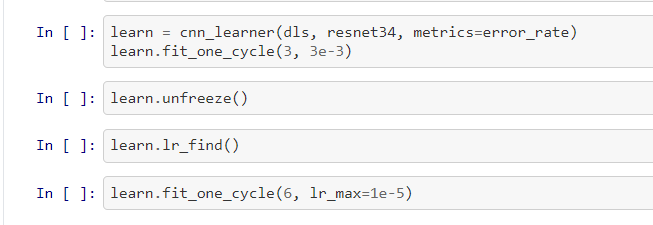• Fastai `fine_tune` method under the hood:
1. Trains randomly for added layers initially for certain epochs
2. Unfreeze all the layers, then train again

### Discriminative learning rate, and rest of portions

• First, train the model with a slow learning rate, and then later train faster. This is the fundamental premise
• Early stopping is a not good method as mentioned in the book below

Before the days of 1cycle training it was very common to save the model at the end of each epoch, and then select whichever model had the best accuracy out of all of the models saved in each epoch. This is known as early stopping. However, this is very unlikely to give you the best answer, because those epochs in the middle occur before the learning rate has had a chance to reach the small values, where it can really find the best result. Therefore, if you find that you have overfitted, what you should do is retrain your model from scratch, and this time select a total number of epochs based on where your previous best results were found.

• Using lr_max, with slice method to train the last layers with a faster speed
• We can improve models with deeper architecture like Resnet 34, 50, 101, 152 etc. There are new techniques now like EfficentNet, Transformers, which can give a better accuracy now.
• Then it’s a good idea to train with half-precision floating points, which speeds up the training process considerably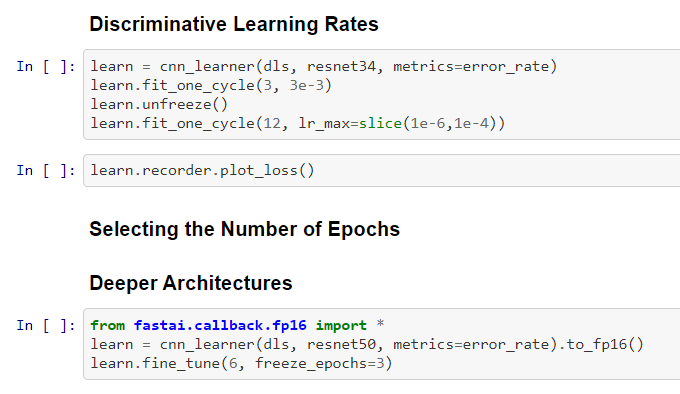Also at the end of the session, @Aman Arora shared four blog post ideas:

1. Write about what `untar_data` method is doing under the hood?
2. Write about what is `fastai foundation class L` is doing. What are the unique features of this special list part of fastai core library?
3. Write about `regex` which is a pretty useful library, and write on what all the cool things you can do with it.
4. Write about `cross_entropy_loss` .

My work logs, trying out things mentioned in Chapter 5 can be found in this Kaggle notebook.

I also got a chance to try out the fastai tool for graphic visualisation `fastdott`, which is pretty cool.